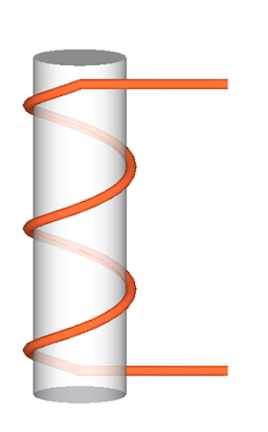# Slide around a Cylinder

Geometry Level 3A slide wraps around a cylinder of radius 3 and height $40\pi$ m exactly 5 times and starts from the top and ends at the bottom. The length of the slide is $x\pi$ m. Find $x$.

Note: In the image, the slide wraps around 2.5 times.

×